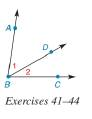Chapter 1.2, Problem 41E### Elementary Geometry for College St...

6th Edition
Daniel C. Alexander + 1 other
ISBN: 9781285195698

#### Solutions

Chapter
Section### Elementary Geometry for College St...

6th Edition
Daniel C. Alexander + 1 other
ISBN: 9781285195698
Textbook Problem
1 views

# In Exercises 41 to 44, m ∠ 1 + m ∠ 2 = m ∠ A B C .Find m ∠ A B C if m ∠ 1 = 32 ° and m ∠ 2 = 39 ° .To determine

To find:

mABC for m1=32° and m2=39°.

Explanation

Calculation:

Given,

m1=32°

m2=39°.

m1+m2=mABC

Substitute; m1

### Still sussing out bartleby?

Check out a sample textbook solution.

See a sample solution

#### The Solution to Your Study Problems

Bartleby provides explanations to thousands of textbook problems written by our experts, many with advanced degrees!

Get Started

#### Find f. f(t) = t + 1/t3, t 0, f(1) = 6

Single Variable Calculus: Early Transcendentals

#### True or False: is a rational function.

Study Guide for Stewart's Single Variable Calculus: Early Transcendentals, 8th

#### Compute 4.8911.34 .

Mathematics For Machine Technology